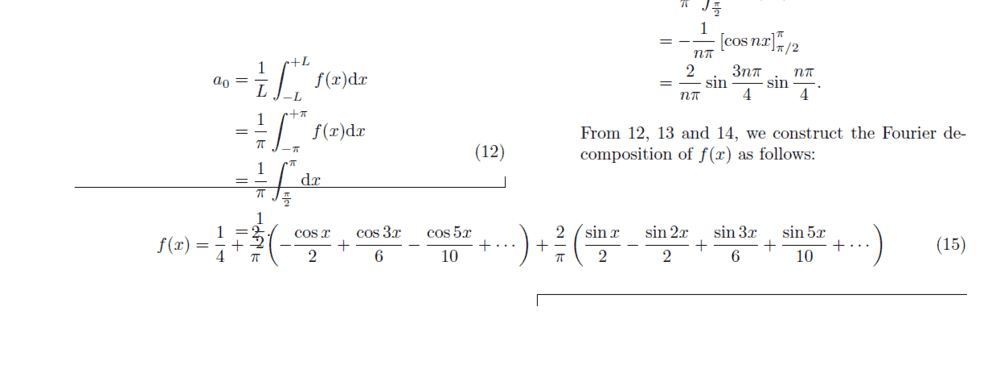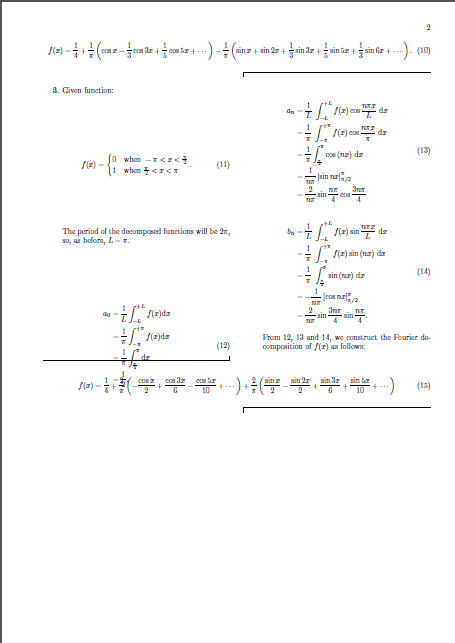# Wide equation in Revtex 4.2 is overlapping with other content

• LaTeX
• Wrichik Basu

#### Wrichik Basu

Gold Member
2022 Award
Our professor has asked us to submit our next assignment in ##\LaTeX##, and he has specifically asked us to use Revtex 4.2. This is not the first time I am working with this document class; in the past, I have used it several times. But I am facing an weird problem this time:This equation was written with the following code:
LaTeX:
\begin{widetext}
\begin{equation}
f(x) = \frac{1}{4} + \frac{2}{\pi}\left(-\frac{\cos{x}}{2} + \frac{\cos{3x}}{6} - \frac{\cos{5x}}{10} + \cdots \right) + \frac{2}{\pi}\left(\frac{\sin{x}}{2} - \frac{\sin{2x}}{2} + \frac{\sin{3x}}{6} + \frac{\sin{5x}}{10} + \cdots \right)
\end{equation}
\end{widetext}
The problem is probably due to the fact that there is not much text content on this page; only equations:Revtex is leaving a lot of gaps between the equations, which is not looking good as well.

I am using the following document class statement:
LaTeX:
\documentclass[reprint, amsmath, amssymb, aps, prl]{revtex4-2}
Any idea how to solve this problem?

The fact that the label (12) is centered between two lines of that equation might be related; perhaps use eqnarray with \nonumber on the first two lines?

The fact that the label (12) is centered between two lines of that equation might be related; perhaps use eqnarray with \nonumber on the first two lines?
This is how eqn. 12 is generated:
LaTeX:
\begin{equation}
\begin{split}
a_0 &= \dfrac{1}{L} \int_{-L}^{+L} f(x) \d x \\
&= \dfrac{1}{\pi} \int_{-\pi}^{+\pi} f(x) \d x \\
&= \dfrac{1}{\pi}\int_{\frac{\pi}{2}}^{\pi} \d x \\
&= \frac{1}{2}.
\end{split}
\label{a0_3}
\end{equation}
where \d is
LaTeX:
\renewcommand{\d}{\mathrm{d}}

Then that's not the issue.

Looking at it more closely, it looks like it's just putting the widetext wherever it happens to occur in the right column, irrespective of whatever is in the left column.

There's a thread on stackexchange which suggests that widetext (via one of its dependencies) is known for corrupting vertical spacing.

•Wrichik Basu
Is there a reason you don't just reformat the equation so it fits in a single column, e.g.,
\begin{align} f(x) =& \frac{1}{4} + \frac{2}{\pi}\left(-\frac{\cos{x}}{2} + \frac{\cos{3x}}{6} - \frac{\cos{5x}}{10} + \dotsb \right) \nonumber \\ & {} + \frac{2}{\pi}\left(\frac{\sin{x}}{2} - \frac{\sin{2x}}{2} + \frac{\sin{3x}}{6} + \frac{\sin{5x}}{10} + \dotsb \right) \end{align} ?

Code:
\begin{align}
f(x) =& \frac{1}{4} + \frac{2}{\pi}\left(-\frac{\cos{x}}{2} + \frac{\cos{3x}}{6} - \frac{\cos{5x}}{10} + \dotsb \right) \nonumber \\
& {} + \frac{2}{\pi}\left(\frac{\sin{x}}{2} - \frac{\sin{2x}}{2} + \frac{\sin{3x}}{6} + \frac{\sin{5x}}{10} + \dotsb \right)
\end{align}

I wanted to use widetext in a paper years ago and my PhD supervisor at the time suggested it would be better to just avoid it and change the notation or formatting of the equation so it would fit in a single column instead. I've never seen a need to use widetext since.

(Also, is the sign in front of the ##\sin 5x## term correct? It seems to alternate everywhere else.)

Is there a reason you don't just reformat the equation so it fits in a single column
Since our instructor is also helping us learn LaTeX, he has asked us to use at least two widetext environments. Otherwise I would have happily formatted the equation to single column, as I have done numerous times before.

By the way, wanted to post an update: the problem has been solved. The solution is nothing special: I just added one MATLAB graph as a picture and the overlapping was gone.

(Also, is the sign in front of the sin⁡5x term correct? It seems to alternate everywhere else.)
Yes, as far as I can see from my analytical calculations and MATLAB. There is a minus sign again for ##\sin{6x}##.

This is how eqn. 12 is generated:
LaTeX:
\begin{equation}
\begin{split}
a_0 &= \dfrac{1}{L} \int_{-L}^{+L} f(x) \d x \\
&= \dfrac{1}{\pi} \int_{-\pi}^{+\pi} f(x) \d x \\
&= \dfrac{1}{\pi}\int_{\frac{\pi}{2}}^{\pi} \d x \\
&= \frac{1}{2}.
\end{split}
\label{a0_3}
\end{equation}
Could it be that it is split that is messing up the spacing? Does the same problem appear if you use eqnarray instead?

Could it be that it is split that is messing up the spacing? Does the same problem appear if you use eqnarray instead?
I haven't tried eqnarray. There is a question on TeX-LaTeX SE on align vs eqnarray, and it has several answers that advise against using this environment. Overleaf showed how to use split instead.

What is your opinion on the use of eqnarray?

Since our instructor is also helping us learn LaTeX, he has asked us to use at least two widetext environments. Otherwise I would have happily formatted the equation to single column, as I have done numerous times before.

Okay, that makes sense.

By the way, wanted to post an update: the problem has been solved. The solution is nothing special: I just added one MATLAB graph as a picture and the overlapping was gone.

I don't know for sure, but it may just have been a problem with RevTeX. Personally I usually avoid using RevTeX when I can since it seems to have trouble with placement of things not unlike what you've seen, particularly when using the pra/prb/etc. options. RevTeX with these options produces slightly more compressed text than it does with the prl option and I wonder if it isn't taking this into account correctly. Conversely, formatting with the prl option generally seems to be okay, but then you lose the section numbers.

I haven't tried eqnarray. There is a question on TeX-LaTeX SE on align vs eqnarray, and it has several answers that advise against using this environment. Overleaf showed how to use split instead.

What is your opinion on the use of eqnarray?

There is a good discussion of this (and typesetting equations in general) in "How to typeset equations in ##\LaTeX##" by Stefan Moser, available here: https://moser-isi.ethz.ch/docs/typeset_equations.pdf.

Short answer: use the IEEEeqnarray environment from the IEEEtrantools package. I am actually surprised more people don't do this since "The Not So Short Introduction to ##\LaTeX2e##" has been recommending this for some years. (It didn't yet when I first started learning LaTeX in the late 2000s.)

•Wrichik Basu
I haven't tried eqnarray. There is a question on TeX-LaTeX SE on align vs eqnarray, and it has several answers that advise against using this environment. Overleaf showed how to use split instead.

What is your opinion on the use of eqnarray?
I usually use align. You may try that as well.

•Wrichik Basu
Submitted the assignment today. I could convince our instructor that using too many widetext environments doesn't look good. I used the align environment as suggested by @wle in post #5 and @DrClaude in post #11, along with the equation environment where necessary. The breqn package has a dmath environment that automatically breaks equations, and also allows breaking \left ... \right pair of delimiters. I eliminated the split environment completely. The figures were creating a problem sometimes, overlapping with text or going below the bottom margin. So I used the geometry package to specify the margins, and that partially solved the problem.

AutoHotkey, suggested by @fresh_42 elsewhere, comes very handy when writing long documents in ##\LaTeX##.